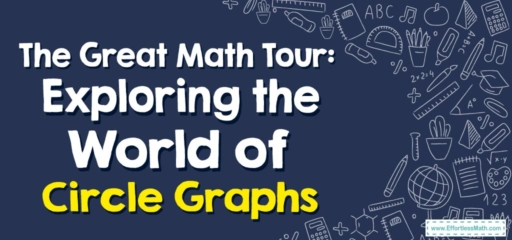# The Great Math Tour: Exploring the World of Circle Graphs

Hello, math explorers!

Today, our math tour takes us to the exciting world of Circle Graphs, also known as Pie Charts. These circular marvels are used to display data in a visually appealing way, making it easier to interpret. So, fasten your seatbelts, and let's set off on our math adventure!## 1. The Destination: Circle Graphs

Circle graphs, much like the delicious pies they are named after, are divided into several ‘slices’ or ‘sectors’. Each slice represents a different category of data, and the size of the slice corresponds to its proportion of the whole.

## 2. The Journey: Interpreting Circle Graphs

Interpreting circle graphs is all about understanding the proportions and percentages that each slice of the pie represents.

### Your Travel Guide: How to Interpret Circle Graphs

Here’s our route:

#### Step 1: Identify the Categories

Firstly, identify the different categories represented in the circle graph. These are usually labeled or explained in a key.

#### Step 2: Understand the Proportions

Each slice of the pie represents a fraction of the whole circle. The larger the slice, the greater the proportion it represents.

#### Step 3: Read the Percentages

Often, circle graphs are labeled with percentages. This tells you what percentage of the whole each category represents.

For instance, if we have a circle graph showing the favorite fruits of a class of students with $$50$$$$\%$$ apples, $$30$$$$\%$$ bananas, and $$20$$$$\%$$ oranges:

1. Identify the Categories: The graph represents students’ preferences for apples, bananas, and oranges.
2. Understand the Proportions: The apple slice is the largest, the banana slice is the next largest, and the orange slice is the smallest.
3. Read the Percentages: Half of the students prefer apples, $$30$$$$\%$$ prefer bananas, and $$20$$$$\%$$ prefer oranges.

And that concludes our math tour to Circle Graphs! I hope you enjoyed the journey and found a new appreciation for these pie-shaped data representations. Remember, the world of math is vast and full of wonder, so keep exploring and discovering. Until our next math adventure!

### What people say about "The Great Math Tour: Exploring the World of Circle Graphs - Effortless Math: We Help Students Learn to LOVE Mathematics"?

No one replied yet.

X
30% OFF

Limited time only!

Save Over 30%

SAVE $5 It was$16.99 now it is \$11.99